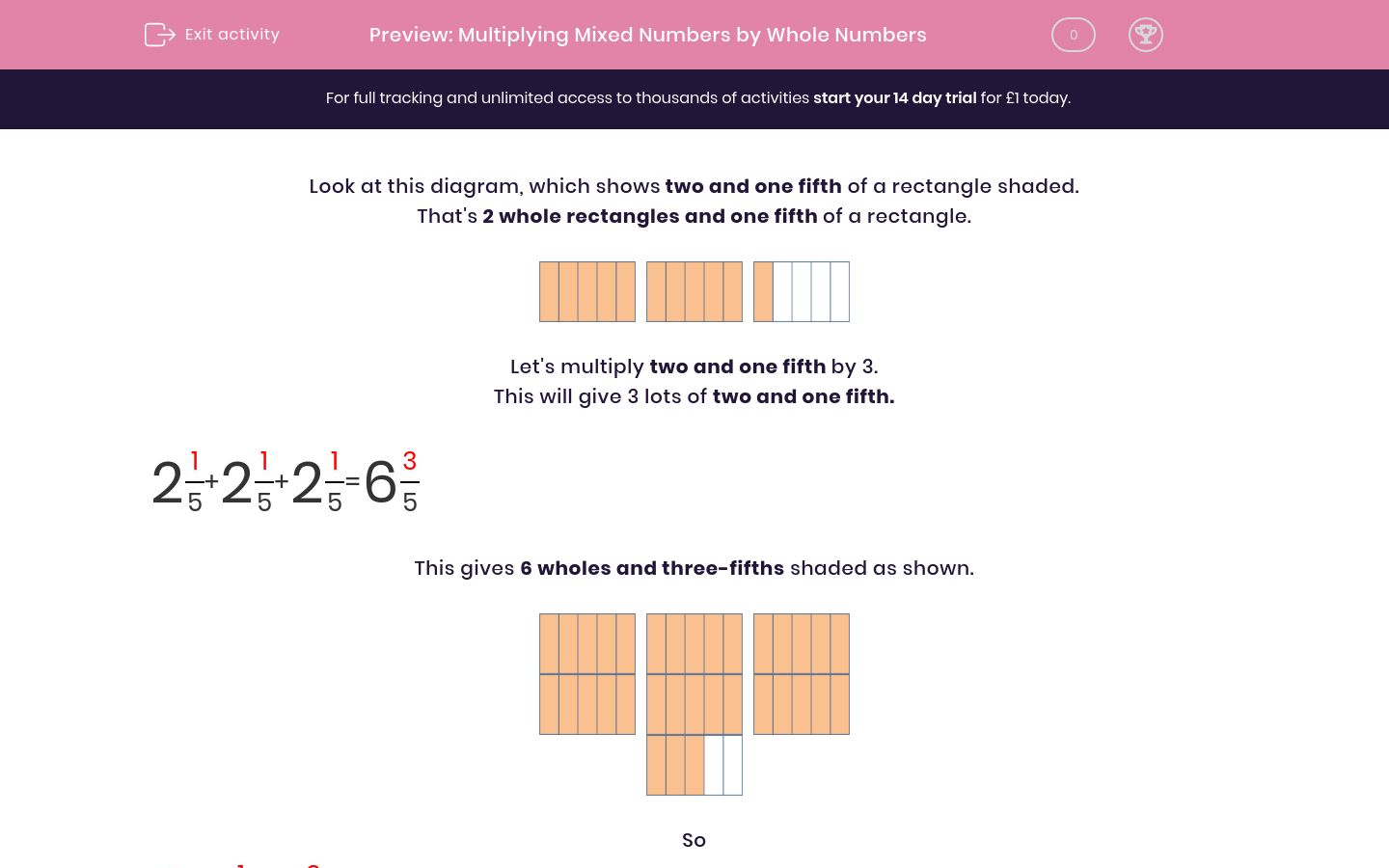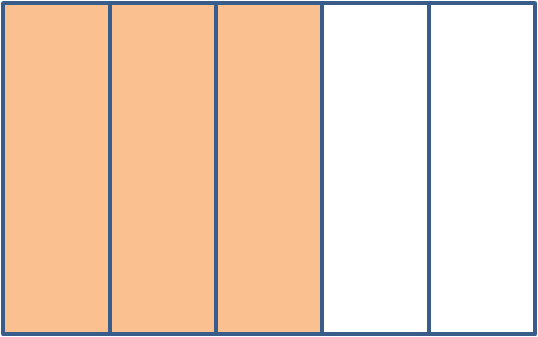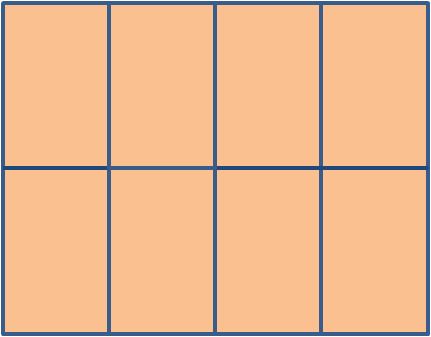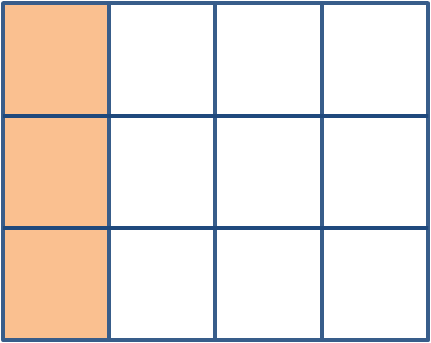# Multiplying Mixed Numbers by Whole Numbers

In this worksheet, students use diagrams to multiply mixed numbers by whole numbers. Answers may involve mixed numbers.Key stage:  KS 2

Curriculum topic:   Maths and Numerical Reasoning

Curriculum subtopic:   Fractions

Difficulty level:### QUESTION 1 of 10

Look at this diagram, which shows two and one fifth of a rectangle shaded.

That's 2 whole rectangles and one fifth of a rectangle.Let's multiply two and one fifth by 3.

This will give 3 lots of two and one fifth.

 2 1 + 2 1 + 2 1 = 6 3 5 5 5 5

This gives 6 wholes and three-fifths shaded as shown.So

 3 × 2 1 = 6 3 5 5Use the diagram to work out and complete:

 3 × 1 1 = 3 ? 4 4

(Just write the missing number shown by the ? mark.)Use the diagram to work out and complete:

 2 × 1 2 = 2 ? 6 6

(Just write the missing number shown by the ? mark.)Use the diagram to work out and complete:

 3 × 1 2 = 3 ? 8 8

(Just write the missing number shown by the ? mark.)Use the diagram to work out and complete:

 3 × 3 2 = ? 6 8 8

(Just write the missing number shown by the ? mark.)Use the diagram to work out and complete:

 3 × 1 3 = 3 9 12 ?

(Just write the missing number shown by the ? mark.)Use the diagram to work out and complete:

 3 × 2 1 = 6 ? 4 4

(Just write the missing number shown by the ? mark.)Use the diagram to work out and complete:

 2 × 3 2 = 6 ? 6 6

(Just write the missing number shown by the ? mark.)Use the diagram to work out and complete:

 5 × 2 1 = 10 ? 6 6

(Just write the missing number shown by the ? mark.)Use the diagram to work out and complete:

 3 × 2 3 = 6 ? 12 12

(Just write the missing number shown by the ? mark.)Use the diagram to work out and complete:

 2 × 2 3 = 4 6 8 ?

(Just write the missing number shown by the ? mark.)

• Question 1Use the diagram to work out and complete:

 3 × 1 1 = 3 ? 4 4

(Just write the missing number shown by the ? mark.)

3
EDDIE SAYS
1/4 + 1/4 + 1/4 = 3/4
• Question 2Use the diagram to work out and complete:

 2 × 1 2 = 2 ? 6 6

(Just write the missing number shown by the ? mark.)

4
EDDIE SAYS
2/6 + 2/6 = 4/6
• Question 3Use the diagram to work out and complete:

 3 × 1 2 = 3 ? 8 8

(Just write the missing number shown by the ? mark.)

6
EDDIE SAYS
2/8 + 2/8 + 2/8 = 6/8
• Question 4Use the diagram to work out and complete:

 3 × 3 2 = ? 6 8 8

(Just write the missing number shown by the ? mark.)

9
EDDIE SAYS
3 × 3 = 9
• Question 5Use the diagram to work out and complete:

 3 × 1 3 = 3 9 12 ?

(Just write the missing number shown by the ? mark.)

12
EDDIE SAYS
The denominator doesn\'t change.
• Question 6Use the diagram to work out and complete:

 3 × 2 1 = 6 ? 4 4

(Just write the missing number shown by the ? mark.)

3
EDDIE SAYS
1/4 + 1/4 + 1/4 = 3/4
• Question 7Use the diagram to work out and complete:

 2 × 3 2 = 6 ? 6 6

(Just write the missing number shown by the ? mark.)

4
EDDIE SAYS
2/6 + 2/6 = 4/6
• Question 8Use the diagram to work out and complete:

 5 × 2 1 = 10 ? 6 6

(Just write the missing number shown by the ? mark.)

5
EDDIE SAYS
1/6 + 1/6 + 1/6 + 1/6 + 1/6 = 5/6
• Question 9Use the diagram to work out and complete:

 3 × 2 3 = 6 ? 12 12

(Just write the missing number shown by the ? mark.)

9
EDDIE SAYS
3/12 + 3/12 + 3/12 = 9/12
• Question 10Use the diagram to work out and complete:

 2 × 2 3 = 4 6 8 ?

(Just write the missing number shown by the ? mark.)

8
EDDIE SAYS
The denominator doesn\'t change.
---- OR ----

Sign up for a £1 trial so you can track and measure your child's progress on this activity.

### What is EdPlace?

We're your National Curriculum aligned online education content provider helping each child succeed in English, maths and science from year 1 to GCSE. With an EdPlace account you’ll be able to track and measure progress, helping each child achieve their best. We build confidence and attainment by personalising each child’s learning at a level that suits them.

Get started